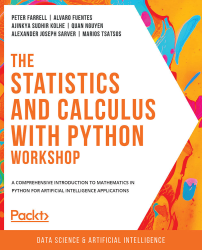•#### The Statistics and Calculus with Python Workshop#### Overview of this book

Are you looking to start developing artificial intelligence applications? Do you need a refresher on key mathematical concepts? Full of engaging practical exercises, The Statistics and Calculus with Python Workshop will show you how to apply your understanding of advanced mathematics in the context of Python. The book begins by giving you a high-level overview of the libraries you'll use while performing statistics with Python. As you progress, you'll perform various mathematical tasks using the Python programming language, such as solving algebraic functions with Python starting with basic functions, and then working through transformations and solving equations. Later chapters in the book will cover statistics and calculus concepts and how to use them to solve problems and gain useful insights. Finally, you'll study differential equations with an emphasis on numerical methods and learn about algorithms that directly calculate values of functions. By the end of this book, you’ll have learned how to apply essential statistics and calculus concepts to develop robust Python applications that solve business challenges.
Preface1. Fundamentals of PythonFree Chapter
2. Python's Main Tools for Statistics3. Python's Statistical Toolbox4. Functions and Algebra with Python5. More Mathematics with Python6. Matrices and Markov Chains with Python7. Doing Basic Statistics with Python8. Foundational Probability Concepts and Their Applications9. Intermediate Statistics with Python10. Foundational Calculus with Python11. More Calculus with Python12. Intermediate Calculus with PythonAppendix# Confidence Intervals

As we saw with the previous simulations, our sample mean can vary from sample to sample. While, in a simulation, we have the luxury of taking 10,000 samples, we cannot do that in the real world; it would be far too expensive and time-consuming. Typically, we are given only enough resources to gather one sample. So how can we be confident in the results of our sample? Is there any way we can account for this variability when reporting our sample mean?

The good news is that the CLT gives us an idea of the variance in our sample mean. We can apply the CLT and take sampling variability into account by using a confidence interval. More generally, a confidence interval is a range of values for a statistic (an example of a statistic is a sample mean) based on a distribution that has some degree of confidence of how likely it is to contain the true value for the mean. We are not always going to be calculating confidence intervals for just the sample mean; the idea applies...